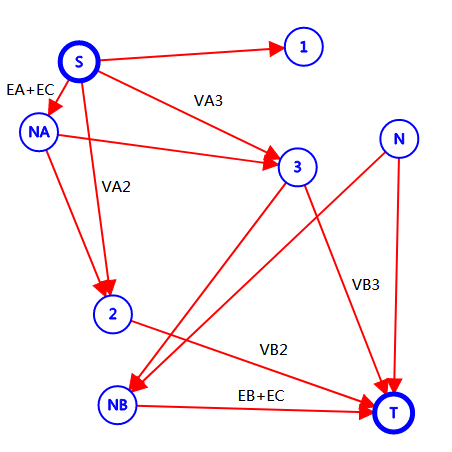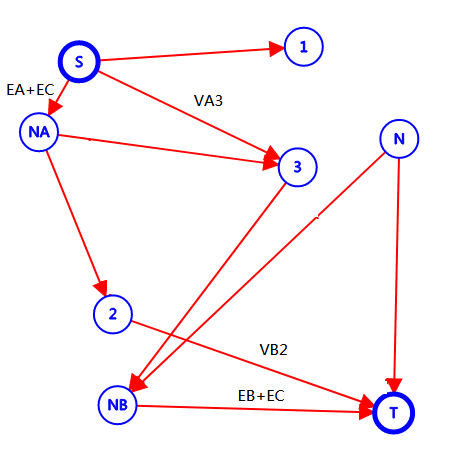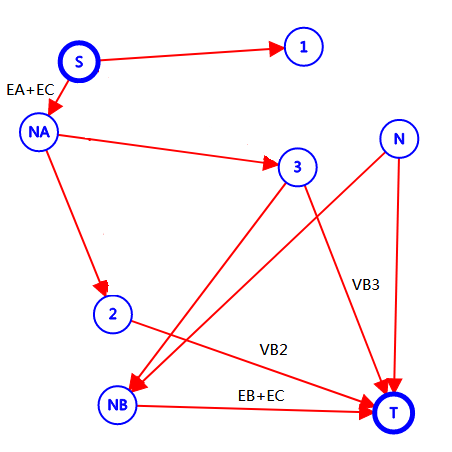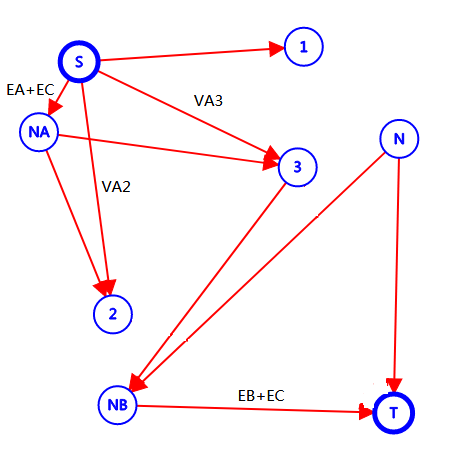# YZOJ P1868 土地划分

• ### 题目描述

1，对于城市 $$i$$ ，如果它划分给 $$A$$ 国，将得到 $$VA[i]$$ 的得分；划分给 $$B$$ 国，将得到 $$VB[i]$$ 的得分。

2，对于一条公路 $$i$$ ，如果它连接两个 $$A$$ 国的城市，将得到 $$EA[i]$$ 的得分；连接两个 $$B$$ 国的城市，将得到 $$EB[i]$$ 的得分；否则，这条公路将失去意义，将扣除 $$EC[i]$$ 的得分。

• ### 数据规模与约定

$$100\%$$ 的数据 $$N \leq 10000, M \leq 40000$$；

Source: BZOJ 3511

$$S$$ 向 $$1$$ 连 $$\infty$$ 的边，$$N$$ 向 $$T$$ 连 $$\infty$$ 的边。

$$S$$ 向 $$i$$ 连 $$VA_i$$ 的边，$$i$$ 向 $$T$$ 连 $$VB_i$$ 的边。（注：图中未注明边权的为 $$\infty$$）